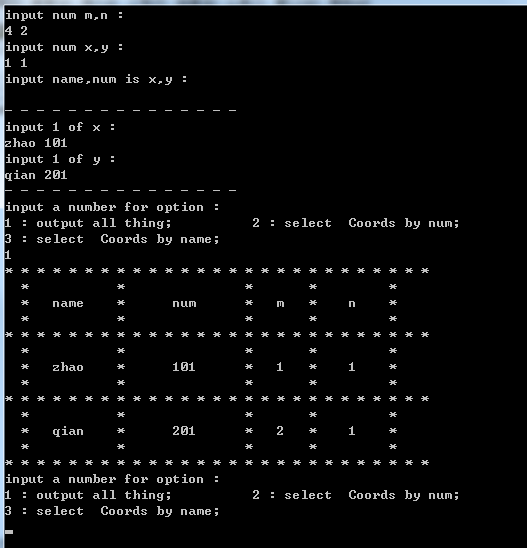2016-12-20 01:16

# 数据结构课程设计完整源代码 用C语言编写

1.按照测验的规则安排座位：
（1）一个教室有m行n列个座位，两个班合堂，学生数分别为x、y，学生学号顺次编号。（2）排座位规则：一列单号一列双号，都是按照学号顺次排列；先排一班再排二班。要求：（1）输出每一个座位上学生学号和姓名； （2）按照学号查一个学生的座位； （3）按照姓名查一个学生的座位。

• 点赞
• 写回答
• 关注问题
• 收藏
• 邀请回答

#### 3条回答默认 最新

•已采纳

这个地方涉及到学生学号，姓名，和座位号；故设计如下结构体：
struct
{
String name;// 学生名字
int num; // 学号
int m; // 行号
int n; // 列号
}student;
定义一个结构体数组，长度是x+y；然后模拟排座位要求，给学生分配作为号：（这里以1班，x人为例）
for(i=1;i<=x;i++){
if(x%2==0){
student[i].name = name;
student[i].num = num;
student[i].m = x/(2*n);
student[i].n = x%n;
}
} 当是2班的是后计算是要给数据加上x,避免覆盖。
然后因为想x,y的不定性，所以要先要求输入x,y和学生的名字和学号；输出则是循环遍历该数组并输出他的每个值。
查找是遍历匹配问题。你没有写，你使用的语言，我就不给你完成代码了，c/c++和java都可以实现。希望对你有用

点赞 评论
•shen_wei 2016-12-20 02:20
`````` struct Student
{
char Name;
char Num;
int nCol;
int nVec;
};

int main()
{
Student Class_1[x] = {0};
Student Class_2[y] = {0};

Student NewClass[x + y] = {0};

void InitStudent(Student stu);

void StudentSort();

void SelectInfo(Student stu);

return 0;
}
``````

一个简要大纲。。

点赞 评论
•这里包含了注释和我写的时候的遇到问题解决方式：
不能发文件，就直接贴代码了，包括注释，先给个图片``````//#include<Cstring>
using namespace std;

#define MAX 100

struct student
{
//  string name;// 学生名字
char name;  // string 有输入方面的问题，我这里改为使用 char*
char  num;  // 学号使用char 便于后边函数计算
int  m; // 行号
int n;  // 列号
};

student stu[MAX]; //这里我是用一定义个最大值，你也可以动态的定义 设置全局变量方便调用

/*
* 输出所有值的函数
* num 长度
*/
void output(int length)
{   // * 的网格标记是给自己计算的，不会计算 就把 所有输出 * 注释，把注释的取消注释；
cout<<"* * * * * * * * * * * * * * * * * * * * * * * * * * *"<<endl;
cout<<"  *           *               *       *         *"<<endl;
cout<<"  *   name    *      num      *   m   *    n    *"<<endl;
cout<<"  *           *               *       *         *"<<endl;
for(int i = 0; i< length; i++)
{
cout<<"* * * * * * * * * * * * * * * * * * * * * * * * * * *"<<endl;
cout<<"  *           *               *       *         *"<<endl;
cout<<"  *   ";
//  cout<<" name : "<<stu[i].name;
cout<<stu[i].name<<"    *      ";

//  cout<<" num : "<<stu[i].num;
cout<<stu[i].num<<"      *   ";

//  cout<<" m : "<<stu[i].m;
cout<<stu[i].m<<"   *    ";

//  cout<<" n : "<<stu[i].n;
cout<<stu[i].n<<"    *"<<endl;
cout<<"  *           *               *       *         *"<<endl;
}
cout<<"* * * * * * * * * * * * * * * * * * * * * * * * * * *"<<endl;
}

/*
* 输出坐标
* type 标志位 标志调用的地方 ch 查找的参数  num 长度
*/
void outputCoords(int type, char *ch, int length)
{
if(type == 1)
{   // type == 1 则ch 表示 num
for(int i = 0; i< length; i++)
{
if(!strcmp(stu[i].num ,ch))
{
cout<<"m : "<<stu[i].m;
cout<<" n : "<<stu[i].n<<endl;
}
}
}
else
{   // type == 0 则 ch 表示 name
for(int i = 0; i< length; i++)
{
if(!strcmp(stu[i].num ,ch))
{
cout<<"m : "<<stu[i].m;
cout<<" n : "<<stu[i].n<<endl;
}
}
}
}

void main(){

int x,y,i,m,n;
//  student stu[MAX]; //这里我是用一定义个最大值，你也可以动态的定义

/*
*关于下面的代码 因为没有明确提及必须输入，所以你也可以在这里直接定义内容（不输入）
*  -- 我习惯输入这里就这么写
*/
cout<<"input num m,n :"<<endl;
cin>>m>>n;
cout<<"input num x,y :"<<endl;
cin>>x>>y;
cout<<"input name,num is x,y :"<<endl;

if(x*y > m*n)
{
cout<<"座位数少许人数"<<endl;
return;
}

cout<<"\n- - - - - - - - - - - - - - -"<<endl; //为了美观：
//录入学生信息 1班
for(i=0;i<x;i++)
{
cout<<"input "<<i+1<<" of x :"<<endl;
cin>>stu[i].name;
cin>>stu[i].num;
}
//录入学生信息 2班
for(i=x;i<(x+y);i++)
{
cout<<"input "<<i-x+1<<" of y :"<<endl;
cin>>stu[i].name;
cin>>stu[i].num;
}   //以上2次可以合并
cout<<"- - - - - - - - - - - - - - -"<<endl;

/*
* 分配座位号
*本次分配假设每个班人数都是偶数个，是给人分座位号，如果考虑到人数为奇数
* 那应该给座位填人的模拟方式 分配
*/
for(i=0;i<(x+y);i++){
int k = i+1;    //实际的基数，偶数
if(k%2==0){
//前边输入过了 name 和 num 这里就不在赋值
stu[i].m = i/(2*n)+2;   //之前给你的赋值，写的着急了，不太准确
stu[i].n = i%(2*n)/2+1;
}
//这一半之前忘记给你写了，这是和上边对称的
if(k%2==1){
stu[i].m = i/(2*n)+1;
stu[i].n = i%(2*n)/2+1;
}
}

/*
* 这里给你说一下确定行列值m，n的思路：
*  1.这里是奇偶数分行，可以2行看做一行，最后分开计算，因为没有第0行所以要加上最小值，抹去零值问题。
*  2.他在当前行的列数实际就是他们对列数N的余数，因为是2行按一行计算，求得结果换回实际值，即除2；记得+1去掉零值。
*/

char ch;
do{
cout<<"input a number for option :"<<endl;
cout<<"1 : output all thing;          2 : select  Coords by num;"<<endl;
cout<<"3 : select  Coords by name;"<<endl;
cin>>i;
switch(i)
{
case 1:output(x+y);break;
case 2:cout<<"input a num : ";
cin>>ch;
outputCoords(1,ch,x+y);
break;

case 3:cout<<"input a name : ";
cin>>ch;
outputCoords(0,ch,x+y);
break;
default:
cout<<" error !";
break;
}

}while(i!=0);
}

``````
点赞 评论